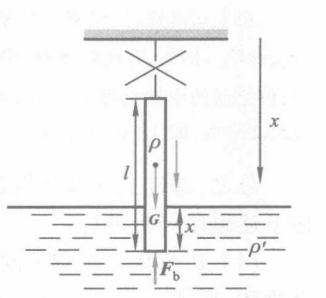$$F=G-F_{\mathrm{b}}=\rho l g-\rho^{\prime} x g$$

$$\left(\rho l-\rho^{\prime} x\right) g=m \frac{\mathrm{d} v}{\mathrm{~d} t}$$

$$\left(\rho l-\rho^{\prime} x\right) g \mathrm{~d} x=m v \mathrm{~d} v$$

$$\int_0^l\left(\rho l-\rho^{\prime} x\right) g \mathrm{~d} x=\int_0^v m v \mathrm{~d} v=\rho l \int_0^v v \mathrm{~d} v$$

$$v=\sqrt{\frac{2 \rho l g-\rho^{\prime} \lg }{\rho}}$$